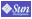Template:Labviewconfigurationkey/doc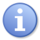This template employs intricate features of template syntax. You are encouraged to familiarise yourself with its setup and parser functions before editing the template. If your edit causes unexpected problems, please undo it quickly, as this template may appear on a large number of pages.Remember that you can conduct experiments, and should test all improvements, in either the general Template sandbox or your user space before changing anything here.

If you want to experiment with the template goto: labviewconfigurationkeysandbox.
For support issues on this template see here.

Usage

To use the template copy and paste the following code as shown below into the editable page.

 {{ labviewconfigurationkey | keyname = | example = | datatype = | description = | permitted_values = | default = | LV1 = | LV2 = | LV3 = | LV4 = | LV5 = | LV6 = | OS1 = | OS2 = | OS3 = | OS4 = | notes = }}

Example

Shown below is an example of the template used with real values inserted.

 {{ labviewconfigurationkey | keyname = copyDeleteFPDCOFromFPTerm | example = copyDeleteFPDCOFromFPTerm=TRUE | datatype = b | description = Delete front panel terminals from diagram | permitted_values = TRUE or FALSE | default = FALSE | LV1 = 3 | LV2 = 4 | LV3 = 5 | LV4 = 6 | LV5 = 7 | LV6 = 8 | OS1 = w | OS2 = m | OS3 = l | OS4 = u | notes = }}

You do not need to include all rows. Any can be omitted. For example, if only one LabVIEW version is supported, the other LV parameters can be removed. You can also leave the parameter in place but not include a value. This may be useful for future edits. The above example produces the following formatted output:

copyDeleteFPDCOFromFPTerm

Example copyDeleteFPDCOFromFPTerm=TRUE Delete front panel terminals from diagram Boolean TRUE or FALSE FALSE 3.x , 4.x , 5.x , 6.x , 7.x , 8.x

The following parameters are explained further below. At any time, you can use a value that is not specified here. It will be accepted as typed. For example, if you want to specify LabVIEW version 6.0.1, then you would use: LV1 = 6.0.1

Parameter Name Options
datatype b=Boolean, i=Integer, s=string, p=path, h=Integer HEX, f=Float
LV1-6 3=3.x, 4=4.x, 5=5.x, 6=6.x, 7=7.x, 8=8.x
OS1-4 w=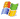, l=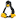, m=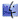, u=